### Home > CALC > Chapter 9 > Lesson 9.1.2 > Problem9-29

9-29.
1. Below is a graph of f(x). Ifthen determine: Homework Help ✎1. F(0)

2. F(1)

3. F' (3)

4. F(6)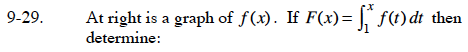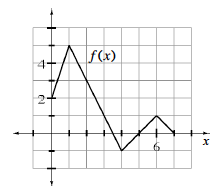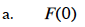$F(0)=\int_1^0f(t)dt$

The integral represents the area under the curve.
Notice that the bounds are reversed.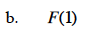$F(1)=\int_1^1f(t)dt$

What is the value of an integral if the bounds are equal?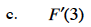$F^\prime(3)=\frac{d}{dx}\int_1^3f(t)dt$

If you evaluate the integral above, will your answer be a number or an expression?
What is the derivative of the number or expression?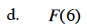What is the area under the curve from x = 1 to x = 6?